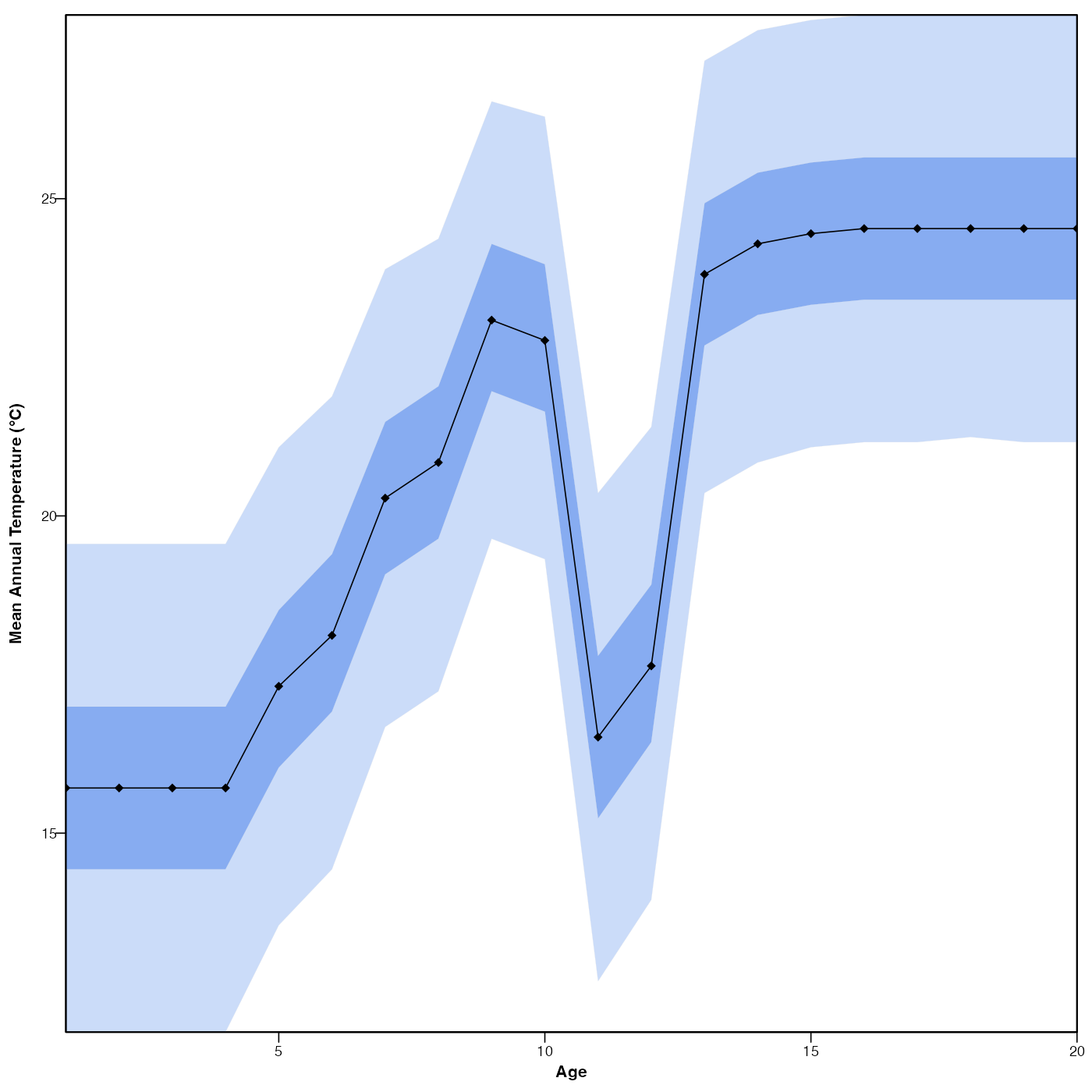Plot the reconstructions and their uncertainties if they exist.

# S3 method for crestObj
plot(
x,
climate = x$parameters$climate,
uncertainties = x$parameters$uncertainties,
optima = TRUE,
simplify = FALSE,
as.anomaly = FALSE,
anomaly.base = x$misc$site_info\$climate[climate],
xlim = NA,
ylim = NA,
pt.cex = 0.8,
pt.lwd = 0.8,
pt.col = ifelse(simplify, "black", "white"),
col.hiatus = "white",
save = FALSE,
width = 5.51,
height = 5.51,
as.png = FALSE,
png.res = 300,
filename = "Reconstruction.pdf",
col = viridis::viridis(125)[26:125],
...
)

## Arguments

x

A crestObj produced by the crest, crest.reconstruct or loo functions.

climate

The climate variables to plot (default is all the reconstructed variables from x)

uncertainties

A (vector of) threshold value(s) indicating the error bars that should be calculated (default are the values stored in x).

optima

A boolean to indicate whether to plot the optimum (TRUE) or the mean (FALSE) estimates.

Adds the modern climate values to the plot.

simplify

A boolean to indicate if the full distribution of uncertainties should be plotted (FALSE, default) or if they should be simplified to the uncertainty range(s).

as.anomaly

A boolean to indicate if the reconstructions should be plotted as absolute values (Default, FALSE) or anomalies '(TRUE).

anomaly.base

The anomaly value. Should be a vector with the same length as climate. Default values are the climate values correpsonding to the location of the record (site_info in crest.get_modern_data).

xlim

the x limits (x1, x2) of the plot. Note that x1 > x2 is allowed and leads to a ‘reversed axis’.

The default value, NULL, indicates that the range of the finite values to be plotted should be used.

ylim

the y limits of the plot.

pt.cex

The size of the points (default 0.8).

pt.lwd

The thickness of the lines (default 0.8).

pt.col

The colour of the points and lines.

col.hiatus

A colour for the hiatus(es) of the record (default white)

save

A boolean to indicate if the diagram should be saved as a pdf file. Default is FALSE.

width, height

The dimensions of the pdf file (default 5.51in ~14cm).

as.png

A boolean to indicate if the output should be saved as a png. Default is FALSE and the figure is saved as a pdf file.

png.res

The resolution of the png file (default 300 pixels per inch).

filename

An absolute or relative path that indicates where the diagram should be saved. Also used to specify the name of the file. Default: the file is saved in the working directory under the name 'Reconstruction_climate.pdf'.

col

...

other graphical parameters (see par and section ‘Details’ below).

## Value

No return value, this function is used to plot.

## Examples

if (FALSE) {
data(crest_ex)
data(crest_ex_pse)
data(crest_ex_selection)
reconstr <- crest(
df = crest_ex, pse = crest_ex_pse, taxaType = 0,
climate = c("bio1", "bio12"), bin_width = c(2, 20),
shape = c("normal", "lognormal"),
selectedTaxa = crest_ex_selection, dbname = "crest_example"
)
reconstr <- loo(reconstr)
}
## example using pre-saved reconstruction obtained with the previous command.
data(reconstr)
plot(reconstr)plot(reconstr, climate='bio1', simplify = TRUE, as.anomaly=TRUE)